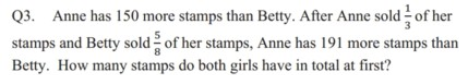# QuestionHow?

Anna                             Betty

At first                        u+150                               u

Sold                          1/3(u+150)                    (5/8)u

Left                           2/3(u+150)                    (3/8)u

Anna has left over 191 more than Betty,

2/3(u+150)(3/8)u = 191    (multiply both sides by 24)

16u +2400 – 9u = 4584

7u = 2184

u = 312

Hence total stamps they have altogether = 2u + 150 = 2(312) + 150 = 774

0 Replies 2 Likes ✔Accepted Answer

Let Betty initially has 24U, 24 because LCM for denominators for the fractions are 3 and 8.

Ann                              Betty

Before             150+24U                     24U

After                2/3  (150+24U)           3/8    (24U)

Given after selling, Ann has 191 stamps more than Betty:

2/3  (150+24U)            =  3/8 (24U) +191

100+16U = 9U +191

7U = 91

U =13

Initially , they have 150 + 48*13=150 +624 =774 stamps together.

0 Replies 1 Like
```Given the fraction 1/3 and 5/8 appearing in the question,
I will typically try using 24u as common unit (because 24 = 3 x 8).

Before selling:
Betty = 24u
Annie = 24u + 150

After selling:
Betty = 3/8 x 24u = 9u
Annie = 2/3 x (24u + 150) = 16u + 100

So difference between Annie and Betty after selling is
16u + 100 - 9u = 7u + 100 = 191. Hence u = 91/7 = 13.

Total number at first is 48u + 150 = 48 x 13 + 150 = 774.

HAPPY LUNAR NEW YEAR EVERYONE!

```

0 Replies 2 Likes